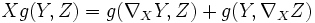# Metric linear connection

## Definition

### Given data

A Riemannian manifold$(M,g)$ (i.e. a differential manifold$M$ endowed with a Riemannian metric$g$).

### Definition part

A metric linear connection on$M$ is a linear connection$\nabla$ on$M$ satisfying the following condition:$X g(Y,Z) = g(\nabla_XY,Z) + g(Y,\nabla_XZ)$

In other words, it is a metric connection on the tangent bundle.

An important case of a metric linear connection is the Levi-Civita connection which is the unique metric torsion-free linear connection.

## Facts

Given a Riemannian manifold$M$, a submanifold$N$, and a metric linear connection on$M$, the induced linear connection on the submanifold$N$ is also a metric connection.

For full proof, refer: Induced connection on submanifold of metric connection is metric